Circuits

# 4. Circuits

Digital systems can be built using a variety of underlying physical technologies: mechanical, chemical, biological, hydraulic, and many others. While many of the lessons of this subject apply to systems built using any physical mechanism, we focus on electronics as our implementation medium. The success of electronics in this (and many other applications) rests on one of the most successful abstractions in the history of engineering: the electronic circuit. In this chapter, we explore the circuit as an abstraction, and review some of its properties as well as its limitations for our purposes.
The Circuit Abstraction

## 4.1. The Circuit Abstraction

A triumph of classical physics is the articulation of Maxwell's equations, shown to the right, which concisely capture the fundamental relationship between electric and magnetic fields.
\begin{equation} \begin{array}{rcl} \nabla\cdot{\bf D} & = & \rho \\ \nabla\cdot{\bf B} & = & 0 \\ \nabla\times{\bf E} & = & - { {\partial{\bf B}}\over{\partial t} } \\ \nabla\times{\bf H} & = & {\bf J} + { {\partial{\bf D} }\over{\partial t} } \end{array} \label{eq:maxwell} \end{equation} Maxwell's Equations
If these equations don't make much sense to you, don't fret: a powerful engineering abstraction offers us ordinary engineers a vastly less complex model, allowing us to design useful electronic systems using simple rules and building blocks. That model is the lumped-element circuit.

Maxwell's equations are admirable for their generality: they apply to arbitrary configurations of continuous 3-space, having arbitrary distributions of physical properties such as electrical conductivity. They consolidate scientific breakthroughs by Faraday, Ampere, Gauss, and other stalwarts of science, and represent a major component of our understanding of classical physics.

As a design space for engineering useful systems, however, they leave much to be desired: their generality, a source of power as a scientific model, gives little guidance to the engineer with a specific problem to solve.

The Lumped-element Circuit

## 4.2. The Lumped-element Circuit

The key to reducing the unconstrained 3-dimensional universe described by Maxwell's Equations to a tractable design space for engineering is to restrict our attention to a tiny subset of possible physical configurations. In particular, instead of considering arbitrary three-dimensional configurations of materials with arbitrary electrical properties, our simplified model considers only finite configurations of discrete components from a small repertoire, electrically isolated from each other except for specific connections made by ideal, perfectly conducting wires.

In this model a circuit is analyzed in terms of voltages and currents, rather than the underlying electric and magnetic fields. A circuit consists of a finite number of components, each having one or more terminals or ports. The ports are connected to equipotential nodes, each consisting of a connected set of ideal wires; at any time, each node carries a single voltage to every port it connects. Current flowing into and out of ports is defined by each component specification in terms of the applied voltages. The constraints of Maxwell's equations are simplified to Kirchoff's laws:

• The sum of all currents entering each node is zero.
• The directed sum of the voltage differences around a cycle is zero.
A common intuitive analogy couches current as the flow of charge, like an incompressable fluid, through the wires and components of a circuit, and voltage as the pressure causing this flow.

This simplified model allows us to define a toolkit of circuit elements that can be connected in various ways, resulting in predictable, intuitive behavior that allows us to address real problems without resorting to Maxwell's equations.

Component Symbol
Battery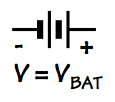switchlampgroundConsider the four circuit elements shown to the right. They include a battery, which acts as a source of a constant voltage; a switch, whose two states prevent or allow current; a lamp, whose resistance $R_{LAMP}$ impedes current flow (but produces light as a side effect), and a ground symbol which identifies a zero-voltage reference point. In terms of the voltages and currents of the circuit model, each of these components has a simple, intuitive behavior that can be used in useful designs by engineers without consideration of the deep physics behind the model. Each of the components applies some local constraint on the voltages on or current through connected nodes, resulting in a set of equations whose solution describes the behavior of the circuit.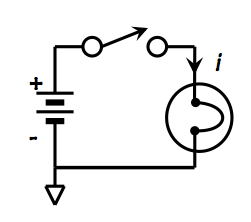To illustrate, consider the simple circuit to the left, representing the electrical components of a typical flashlight. The semantics of this diagram dictates a simple behavior: when the switch is off (open), no current flows and the lamp is dark; when the switch is on (closed), the voltage $V_{BAT}$ applied to the lamp causes a current $i = V_{BAT} / R_{LAMP}$ to flow through the lamp, causing it to emit light. The circuit gives no hint as to the physical location of the components or the wires connecting them: it abstracts away the notion of space from the designer's awareness. In contrast, an analysis of our flashlight at the level of Maxwell's equations would take all of this detail -- and much more -- into account. It would consider the complex of electric and magnetic fields surrounding each component and wire -- details which are physically more realistic, but inconsequential in our application.

We get away with this drastic reduction in design complexity partly because we have developed technology that conforms closely to the ideals of our model. Metal wires are vastly more conductive than insulators, including empty space. Although there are infinitely many physical arrangements of wires and the three components of our flashlight, differing in their analysis at the level of physics, in our application domain they are all equivalent to a very good first approximation.

Linear Circuits

## 4.3. Linear Circuits

It is often useful to view a circuit as taking a time-varying signal -- e.g., a voltage waveform -- applied to some input node, and responding via another time varying signal at an output node. In this view, a circuit C transforms an input waveform given by some time-varying function $f(t)$ into an output waveform $g(t)$; more concisely, $C(f(t)) = g(t)$. The important special case of linear circuits has the property that, for every linear circuit $C$, if $C(f_1(t))=g_1(t)$ and $C(f_2(t))=g_2(t)$ then $C(f_1(t)+f_2(t)) = g_1(t)+g_2(t)$. Linear circuits have been extensively studied, and there is a powerful set of engineering tools for analyzing their behavior,

Although for the construction of digital systems our primary technology is nonlinear circuits, an intuitive appreciation of basic linear components is useful for modeling electrical issues in real-world systems, and understanding the limits of the circuit model.

Linear Circuit Components
Component Symbol v/i
constraint
Resistor$v = R \cdot i$
Capacitor$i = C\cdot {dv \over dt}$
Inductor$v = L\cdot {di \over dt}$
Voltage
Source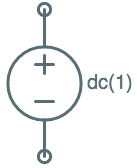$v = fn(t)$
The basic linear circuit components are summarized to the right, along with their symbols and the voltage/current constraint each imposes on the nodes to which it connects. Each of these components is parameterized by a component-specific specification.

A resistor creates a voltage drop between its two ports proportional to the current flowing between them, in accordance with Ohm's law: $v = i \cdot R$. It is called a resistor because the voltage drop generated by the current tends to impede that current; it is analogous to the backpressure generated by flow through a constricted pipe. The resistance $R$ is measured in Ohms.

A capacitor conducts current in proportion to the rate of change in the voltage drop across its terminals; if a constant voltage is applied, no current flows. The constant of proportionality is the capacitance $C$, measured in Farads. A capacitor can be viewed as an energy storage device; indeed, some electronic devices (like digital watches) are powered by capacitors rather than batteries. The energy stored in a capacitor is $C\cdot v^2/2$, where $v$ is the voltage drop across its terminals.

The voltage drop across an inductor is proportional to the rate of change of the current flowing through it; here the constant of proportionality is its inductance, measured in Henrys.

The last component in our linear toolkit is a voltage source, an abstraction we use to mimic batteries and other real-world sources of electrical stimuli. The parameter may be a constant voltage for "DC" or direct current, or may be a time-varying waveform such as a step function or a sinusoid.

Parasitic RLC elements

### 4.3.1. Parasitic RLC elements

The set of components in a circuit implies a set of equations dictating constraints on the voltages and currents throughout that circuit, and analysis of the circuit's behavior typically involves solving these equations. In circuits involving capacitors and inductors, the equations involve derivatives, leading to differential equations to be solved. While the time-domain analysis of arbitrary linear circuits is beyond the scope of this subject, a modest intuitive appreciation of the behavior of linear elements is useful to understand the impact of parasitic resistances, inductances, and capacitances that inevitably creep into the digital circuits we will build.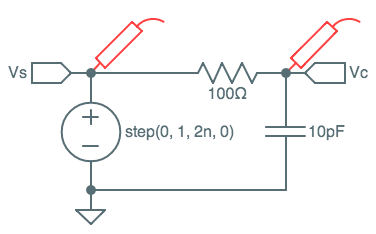RC Circuit
Consider, for example, the simple circuit shown to the right, involving a voltage source driving a step function $V_S$ on a node connected through a resistor and a capacitor to ground. The voltage source serves as a model for the desired behavior at an output node of a circuit we build: we would like the voltage on the output node to change instantaneously from $0v$ to $1v$ at a prescribed time. While we can approximate this idealized behavior in real-world circuits, however, we cannot replicate it perfectly: metal wires have low, but not zero resistance; and the proximity between wires leads to small but consequential capacitive effects as well. We model these parasitic effects in our circuit using an explicit resistor and capacitor, as shown in the diagram.RC Circuit Analysis
The time-domain behavior of this simple circuit is shown to the left: the top waveform shows the step function voltage at the circuit node labeled $V_S$, and the bottom waveform shows the voltage drop across the capacitor. The equations dictated by our components are $i = C\cdot{d{V_C(t)}\over dt}$ and $i = (V_S(t) - V_C(t))/R$, and their solution gives $V_C = 1-e^{-{t/{RC}}}$, an exponential whose value decays toward the 1-volt equilibrium with a time constant inversely proportional to the RC product.
Power and Energy

### 4.3.2. Power and Energy

The instaneous power, in watts, flowing into or out of a two-terminal device is $P = I\cdot V$, where $I$ is the current flowing through the device and $V$ is the voltage drop across its terminals. Power is a measure of the rate of flow of energy; one watt of power corresponds to a flow rate of one joule of energy per second.

In some applications, power may be viewed as an asset: if we were building machines to do mechanical work or cook pizza, we might try to maximize power parameters. In machines designed to process information, however, we usually seek to minimize power flow; power represents energy consumption, and power dissipation generates heat which must be removed by cooling apparatus. In modern computing circuitry, energy consumption has emerged as one of the major bottlenecks among the cost/performance tradeoffs faced by the engineer.

Among our linear circuit elements, the resistor dissipates power by turning it into heat, a form of energy that is unrecoverable for further use by our circuits. Modern digital circuits tend to avoid resistors to minimize their consequent ohmic losses: a voltage drop of $V_R$ across an $R$-ohm resistor causes a current $I_R={V_R \over R}$ and, by Ohm's law, a power dissipation of ${V_R}^2\over R$. Even when we avoid deliberate incorporation of resistors in our digital designs, parasitic resistances (e.g. of wires) dissipate power through ohmic losses wherever current flows in our circuits.

The so-called reactive linear components, capacitors and inductors, do not dissipate power in our circuit model. Rather, the $V\cdot I$ energy pumped in or out of them effects the storage of energy within the device, in the form of an electric (capacitor) or magnetic (inductor) field. The energy stored in a $C$-farad capacitor charged to a voltage of $V$ is ${C\cdot V^2}/2$; the energy stored in an $L$-henry inductor carrying a current $I$ is ${L\cdot I^2}/2$. Although this energy is stored rather than dissipated, in simple digital circuits it is ultimately consumed by ohmic losses in parasitic resistances and consequently wasted. The stored energy is, however, in theory recoverable; and clever circuit designs ("charge recovery circuits") attempt to minimize power dissipation by recycling some of the stored energy.

Analog video toolkit

## 4.4. Analog video toolkit

The productivity of engineering within a particular application domain is enhanced by building a repertoire of reusable building blocks -- a toolkit of generally useful modules for that domain. Ideally, there is a simple, easy to understand conceptual model underlying the toolkit: modules can be plugged together simply to make systems that behave in predictable and useful ways. The design of such toolkits, and their simplicity and coherence, often involves conventions for representing domain-specific information communicated between connected modules of a system.

Consider, for example, a toolkit involving modules for the processing of streams of video, using a plausible "analog" scheme for encoding monochrome video as a continuous voltage waveform. We begin by choosing a convention for encoding the intensity of a single point in an image -- say, 0 volts for black, 1.0 volts for white, and any voltage between 0 and 1 volt to represent the corresponding intermediate shade of gray.Raster Scan
Next, we establish a convention for scanning some subset of the points in an image in some prescribed order -- for example, a left-to-right, top-to-bottom raster scan as shown to the right. We must choose some finite number of horizontal scan lines as part of this encoding scheme, implying that we are ignoring information content of the image between scan lines: our encoding will represent only an approximation of the image obtained by subsampling. Of course, we can choose the number of scan lines (resolution) of the encoding to improve the approximation to some acceptable level.

One complete top-to-bottom scan of the image will generate a voltage waveform that represents, in some finite time interval, the black/white intensities of each point on the raster scan. By repeatedly scanning the image (say, 60 times per second), our waveform will convey consecutive snapshots of the image -- conveying approximate representations of moving images. In fact, this scheme is roughly that used in early analog television.

Suppose we next develop a library of modules for processing this representation of video. One might imagine a video processing laboratory having a wide range of processing blocks to do image enhancement, picture-in-picture compositing, green-screen overlays, and other such building blocks;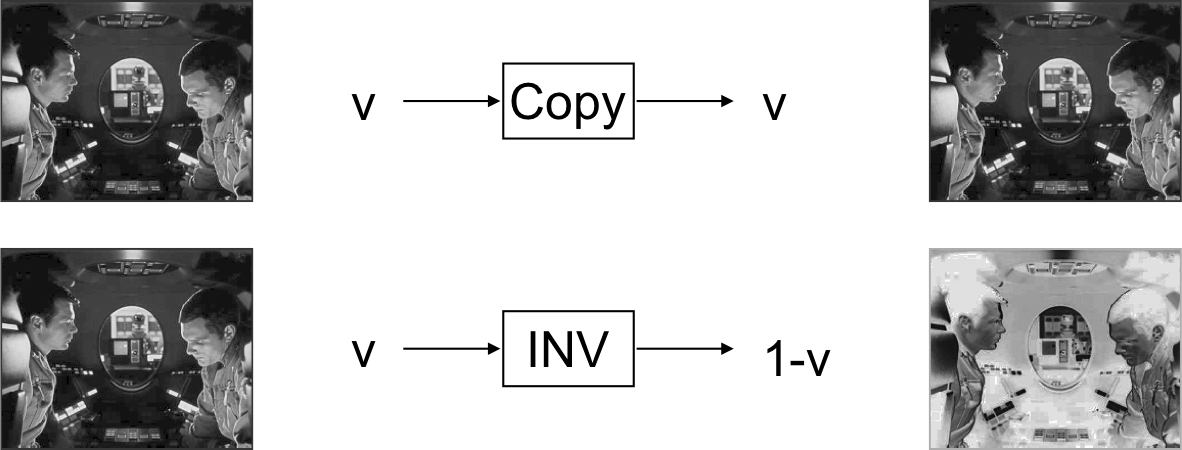Video Operators
to make our point, however, we'll reduce the set of modules to the two shown on the left. The first of these simply copies the video represented at its input to its output, unchanged. It can be viewed as the abstraction of a transmission or storage operation, that moves the video information in space or time. The ideal version of this operator is easy to describe: for any input voltage $V$, it produces the identical $V$ at its output. The second module is slightly more interesting: it inverts the black/white intensity level. Using our voltage encoding, for every input voltage $V$ this module will produce $1-V$ at its output. Observe that two cascaded inversion modules will leave the video unchanged, like our copy module; indeed, any even number of inversions results in unchanged video.

Our simple analog representation of video and tiny repertoire of processing modules constitutes an appealing engineering abstraction. Both the video representation and the voltage-domain description of module behavior may be viewed as idealized, in the sense that they constitute descriptions of perfect, information-preserving building blocks. The descriptions are simple, and it is easy to go into the laboratory and construct actual hardware modules that approximate the voltage-domain behavior of these ideals quite closely.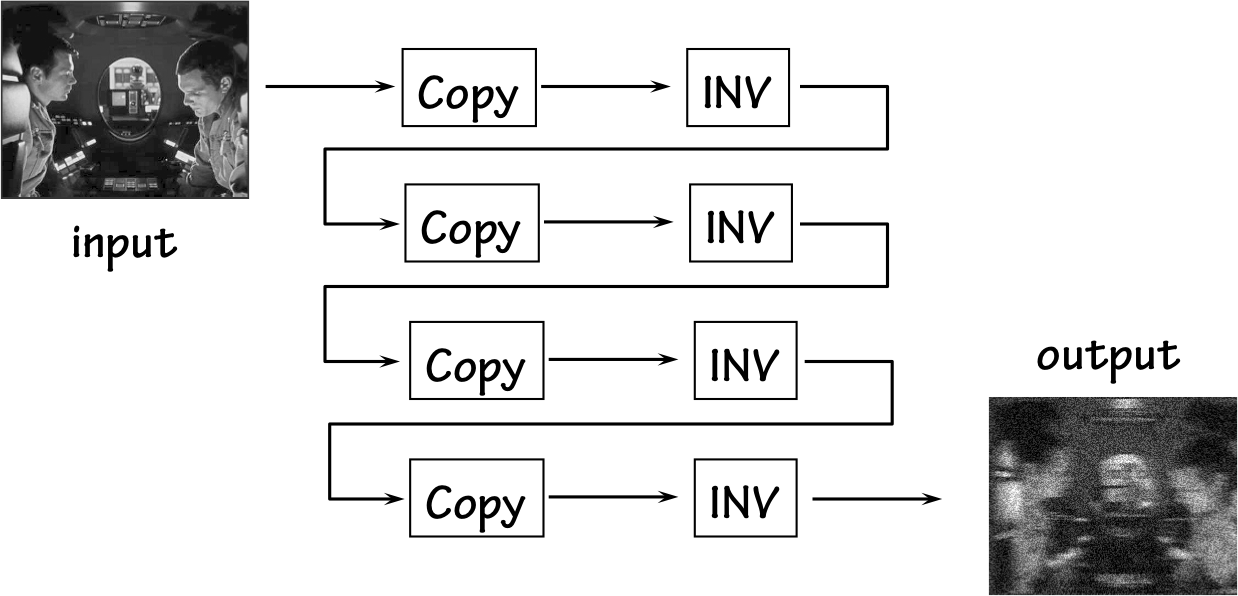Analog Video System
Suppose we build real-world modules and connect them in a system like that shown on the right. Given that the module cascade contains an even number of inversions, our model predicts that the output video will be identical to the input. However, in practice, it will have degraded somewhat from this ideal: inevitably, the output voltage of each module will not be exactly that predicted by our model.

Is this failing of our analog abstraction fatal? The answer is clearly no: analog abstractions have played (and continue to play) an important role in engineering. They do, however, suffer from a major deficiency that has motivated a shift to digital models in many areas of engineering: they describe ideal behavior, and that ideal is unattainable in practice. We cannot insist that a real-world inversion operator literally outputs exactly $1-V$ for input $V$. Then what can we expect from a real-world operator? We can expect some approximation of the ideal behavior, but what approximation?

We can try to address this issue by building some error tolerance into our analog model. We might, for example, specify that the output of each module must be within 1% of its ideal value. Such steps complicate the model, and reduce the appeal of its original elegant simplicity: now, every node of our multi-module system will carry a differently-degraded version of our image.

In Chapter 5 we will develop an engineering abstraction that allows us to build systems which offer some immunity to this form of signal degradation. In the following section, we examine more closely the sources of such problems in our circuit model.

Circuit model limitations

## 4.5. Circuit model limitations

The lumped-element circuit model, and its extension to digital circuits introduced in Chapter 5, represent powerful engineering abstractions responsible for much of our technological progress during recent decades. However, the circumspect circuit designer is keenly aware of physical realities that are ignored by the circuit model in the interests of design simplicity, and adopts engineering disciplines carefully designed to deal with these limitations of the model. In this section, we briefly discuss two classes of circuit model limitations and ways in which we will accommodate them in our engineering practice.
Noise Sources

### 4.5.1. Noise Sources

The first class of circuit model limitations stems from the fact that circuit element specifications represent idealized behavior that can only be approximated by our real-world circuits. Discrepancies between actual and ideal circuit behavior that fall into this category include
• Parisitic resistance, inductance, capacitance, stemming from our inability to tease these electrical effects apart as cleanly as our circuit model dictates;
• Imprecision in parametric values of real-world components, due to manufacturing variations and allowable tolerances;
• Environmental effects such as external magnetic and electric fields, temperature variation, etc.
These and similar inaccuracies in the predictions made by our circuit model, although real, can typically be kept within reasonable bounds by good engineering practice. Accordingly, we will deal with them formally by sweeping them all under a single carpet: we treat them as noise.

More specifically, we will assume that signals the real-world implementations of our circuits are contaminated by some amount of random noise, which appears as a bounded random variable added to the values of voltages and/or currents flowing in our circuits. In analog circuits, this noise component will manifest itself as imperfections in the circuit output (as we saw in Section 4.4). Our digital circuit model, however, will be specifically engineered to tolerate bounded noise without impacting output quality.

Wire Delays

### 4.5.2. Wire Delays

A more serious limitation of the circuit model is the fact that it is oblivious to the physical notion of space. A ten-mile wire has the same circuit-theoretic properties as a one-micron wire: it has a single potential, which can change instaneously and synchronously over its entire length.

We recognize that this model is physically unrealistic for a variety of reasons. Firstly, instantaneous discontinuities in voltage and current are infeasible due to parasitic capacitative and inductive effects: an instantaneous change would require an infinite voltage and/or current spike. But more importantly, we know that a signal takes finite time to propagate from one physical location to another: physics places an inviolable upper bound -- the speed of light -- on signal propagation, and under practical circumstances the effective bound is lower. While the speed of light seems (and is) a generous speed limit for signal propagation, its not infinite. Indeed, the speed of light is on the order of one foot per nanosecond; in the era of high-speed computing, a nanosecond has become a long time.

Historically, the space-oblivious circuit model arose under circumstances where devices were slow relative to signal propagation over the distances involved, and this relation still applies in many applications (such as the flashlight of Section 4.2). However, the assumption of negligible signal transit time is much less applicable to modern, high-speed digital circuits, causing a real tension between the circuit model and our engineering needs.

This tension results in large part from our desire to concisely and locally characterize the behavior of system components, as we did with those of our video toolkit of Section 4.4. We would like, for example, the device specifications to include the timing and values of voltages on the output terminals of a device, and allow the engineer to assume that these are the values and timing that will be seen at inputs connected to these terminals. Unfortunately, the real-world implementation of our device does not have absolute control over the voltage on an output terminal: its actual voltage and timing depends on the loading imposed by the connected circuitry. Worse, that loading includes parasitic effects that depend on the length and routing of connected wires, details that are typically determined very late in the design process.

Our very real dilemma is this: we'd like to give our engineers the Tinkertoy-set simplicity of modules that completely specify their outputs in terms of their inputs. However, physical realities dictate that some aspects of our inputs will be determined by factors (output loading and wire routing) external to the modules, and unknown at the time in the design process when the device is selected.

Fortunately, there is an imperfect but tolerable compromise we can use to address this dilemma. Our tecnology of choice for digital circuits -- CMOS, detailed in Chapter 6 -- has properties that allow us to model the effects of output loading as a simple (unknown) additional delay applied to signals at component output terminals. We can exploit this feature of our technology by the following system design approach:

1. devise an engineering discipline (and associated circuit technology) by which we build sytems with a single system-wide parameter -- clock period -- which dictates both the performance of the system and timing allowed for signals at every node in our circuit.
2. specify the voltage and timing at component outputs assuming a light load, ignoring additional load-dependent delays.
3. Design each system using the optimistic timing of device specifications, understanding that the actual system will run somewhat slower than calculated due to unaccounted-for wire delays. Given the engineering discipline of 1. above, we are assured that for some clock period, the system as designed will work properly.
4. Once a system design is complete, route the wires (a CPU-intensive process) and analyze the actual loading on each circuit node. We use this data to determine the actual clock period (and hence performance) of our system.
A frustrating aspect of this process is the actual performance of a design is not known until the component layout and routing of wires has been completed. Often the process is iterated, trying different design choices and exploring the resulting performance impact due to wire delays. Experienced designers develop good intuition regarding this process; it is one of the aspects of circuit design that is as much an art as a science.

It is worth noting that once a module is designed and its component layout and wire routing have been optimized, the wire delays internal to that component may be incorporated into its specified timing. Module libraries ("cell libraries") typically contain patterns of wires and components that serve as pre-wired templates that can be stamped out for new instances that share the same internal wiring delays.

Chapter Summary

## 4.6. Chapter Summary

The lumped-element circuit is a major engineering abstraction: it reduces the vastly complex range of possible physical systems to a simple, tractible set of functional special cases.

Noteworthy points:

• A circuit consistes of a finite number of circuit elements, each with finitely many terminals;
• Terminals are interconnected via a finite number of equipotential circuit nodes;
• Manufacturable devices provide good approximations of circuit elements and wires provide good approximations of equipotential nodes, under many circumstances; BUT
• The circuit-theoretic ideal ignores inevitable real-world issues such as wire delay, parasitics, and noise.
Many useful complex systems have been built using the lumped circuit model: the video toolkit of Section 4.4 provides a simple taste. As that example illustrates, the circuit provides only an approximate model for the actual system's behavior: to promote device specifications to the level of enforcible contracts, additional engineering disciplines are needed. These are the subject of the next several chapters.